# 年轻人不讲武德，乱用索引，你到底走了多少弯路？1.索引失效常见原因：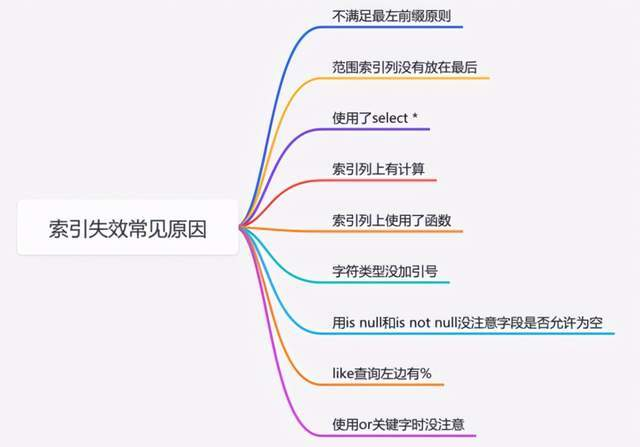2.索引失效常见误区：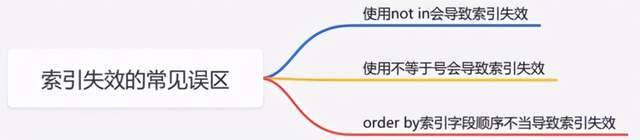3.索引设计的几个建议：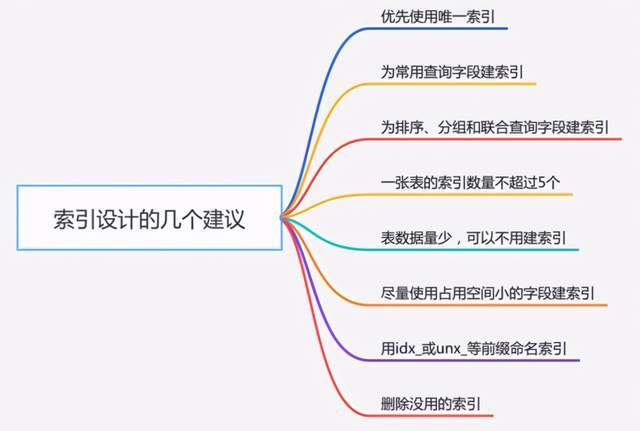# 准备工作

select  VERSION();

CREATE TABLE test1 (  id bigint NOT NULL,  code varchar(30) NOT NULL,  age int NOT NULL,  name varchar(30) NOT NULL,  height int NOT NULL,  PRIMARY KEY (id),  KEY idx_code_age_name (code,age,name) USING BTREE,  KEY idx_height (height) USING BTREE) ENGINE=InnoDB DEFAULT CHARSET=utf8

INSERT INTO test1(id, code, name, age,address) VALUES (1, '001', '张飞', 18,'7');INSERT INTO test1(id, code, name, age,address) VALUES (2, '002', '关羽', 19,'8');

select * from test1;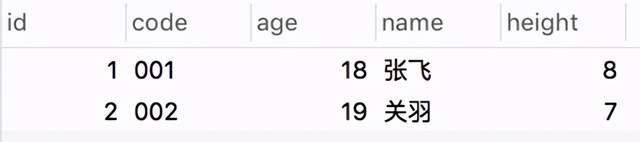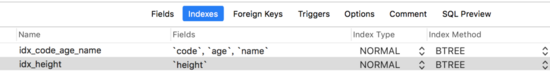# 第 1 种情况：

where 条件后的字段包含了联合索引的所有索引字段，并且顺序是按照： code 、 age 、name 。

explain  select * from test1     where code='001' and age=18  and  name='张飞' ;

# 第 2 种情况：

where 条件后的字段包含了联合索引的所有索引字段，顺序是不按照： code 、 age 、name。

explain  select * from test1     where code='001' and  name='张飞' and age=18;

# 第 3 种情况：

where 条件后的字段包含了联合索引中的： code 字段

explain  select * from test1     where code='001';

# 第 4 种情况：

where 条件后的字段包含了联合索引中的： age 字段

explain  select * from test1     where age=18;

# 第 5 种情况：

where 条件后的字段包含了联合索引中的： name 字段

explain  select * from test1     where  name='张飞';

# 第 6 种情况：

where 条件后的字段包含了联合索引中的： code 和 age 字段

explain  select * from test1    where code='001' and age=18;

# 第 7 种情况：

where 条件后的字段包含了联合索引中的： code 和 name 字段

explain  select * from test1    where code='001' and  name='张飞';

# 第 8 种情况：

where 条件后的字段包含了联合索引中的： age 和 name 字段

explain  select * from test1    where age=18  and  name='张飞';

# 小结：

1. code code、age code、age、name

2. code age name

3. 如果中间出现断层，如： code、name ，只会走第一个索引 code，从断层后的索引都会失效。

4. age name age,name

# 2.范围索引列没有放在最后

where 条件后的字段 age 用了大于等于，具体 sql 如下：

EXPLAIN  select * from test1 where  code='001' and age>18  and  name='张飞' ;

EXPLAIN  select * from test1 where code='001' and  name='张飞' and age>18 ;

# 这是一个非常经典的错误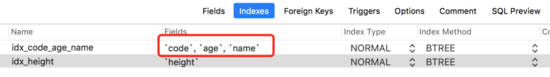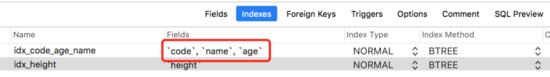EXPLAIN  select * from test1 where  code='001' and age>18  and  name='张飞';

# 3.使用了 select *

EXPLAIN  select * from test1

EXPLAIN  select code,age,name  from test1

# 4.索引列上有计算

explain  select * from test1 where  height+1 =7;

# 5.索引列上使用了函数

explain  select * from test1 where   SUBSTR(height,1,1)=8;

# 6.字符类型没加引号

explain  select * from test1 where  name = 123;

# 7.用 is null 和 is not null 没注意字段是否允许为空

前面创建的test1表中height字段是非空的。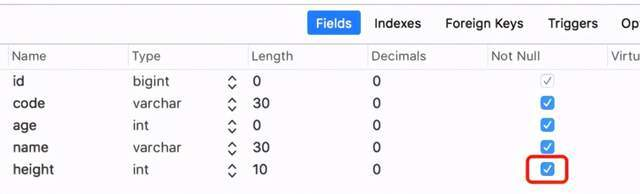explain  select * from test1 where  height is  null;

explain  select * from test1 where  height is  not  null;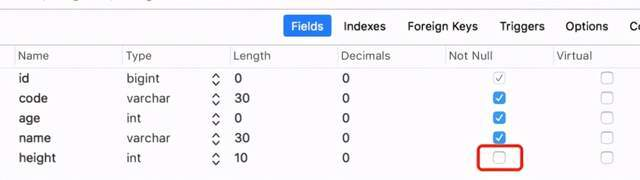# 小结

1. 如果字段不允许为空，则 is null 和 is not null 这两种情况索引都会失效。

2. 如果字段允许为空，则 is null 走 ref 类型的索引，而 is not null 走 range 类型的索引。

# 8.like 查询左边有 %

like 查询主要有三种情况：

• like '%a'

• like 'a%'

• like '%a%'

explain  select * from test1 where  code like '%001';

explain  select * from test1 where  code like  '001%';

explain  select * from test1 where  code like  '%001%';

explain  select code,age,name  from test1 where  code like  '%001%';

• like '%a' 索引失效

• like 'a%' 走 range 类型索引

• like '%a%' 索引失效

# 9.使用 or 关键字时没有注意

用法如下：

explain  select * from test1 where  height = 8  or height = 9;

explain  select * from test1 where  code = '001' or height = 8;

explain  select * from test1 where  code = '001';

explain  select * from test1 where  height = 8;

explain (select * from test1 where   code = '001') union (select * from test1 where  height = 8);

or 关键字会让索引失效，可以用 union 代替

# 1.使用 not in 会导致索引失效

explain  select * from test1 where  height not  in (7,8);

# 2.使用不等于号会导致索引失效

explain  select * from test1 where height!=8;

# 3.order by 索引字段顺序不当导致索引失效

sql 中除了 where 后面的字段能走索引之外， order by 后面的字段也能走索引。

EXPLAIN  select * from test1 where  code='001' order  by age,name;

EXPLAIN  select * from test1 	where  code='001' order  by  name;

EXPLAIN  select * from test1 	where  code='001' order  by  name,age;

# 三. 索引设计的几个建议：

1. 优先使用唯一索引，能够快速定位

2. 为常用查询字段建索引

3. 为排序、分组和联合查询字段建索引

4. 一张表的索引数量不超过 5 个

5. 表数据量少，可以不用建索引

6. 尽量使用占用空间小的字段建索引

7. 用 idx_或 unx_等前缀命名索引，方面查找

8. 删除没用的索引，因为它会占一定空间

# 四. 彩蛋：

• 全职匹配我最爱，最左前缀要遵守

• 带头大哥不能死，中间兄弟不能断

• 索引列上少计算，范围列后全失效

• like 百分写最右，覆盖索引不写*

• 不等空值还有 or ， 索引影响要注意；

• 字符字段引号不能丢，sql 优化有诀窍。

# 最后说一句(求关注，别白嫖我)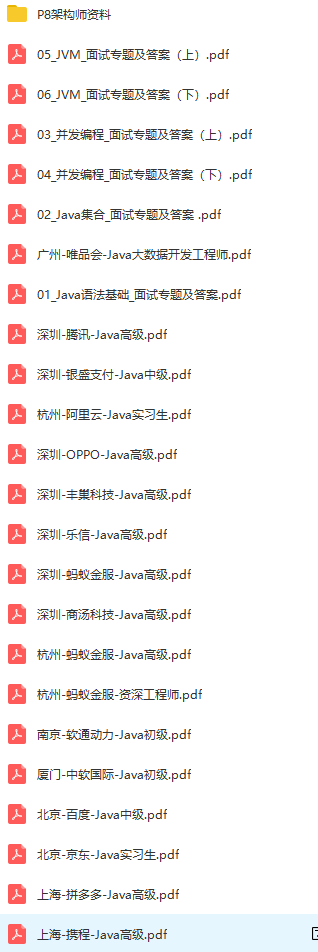## 评论